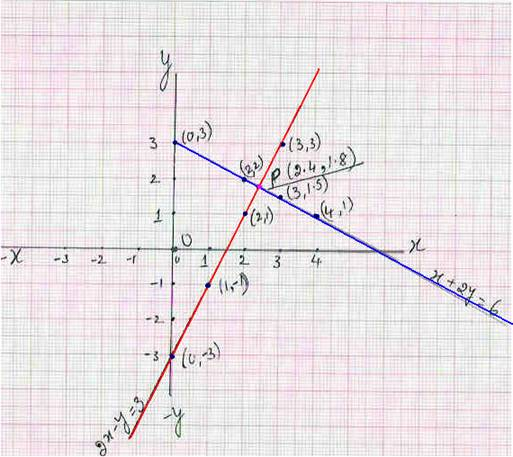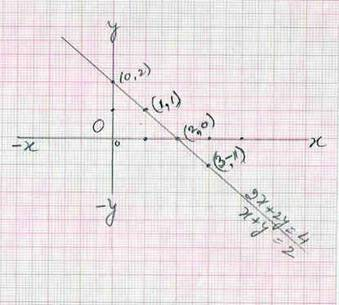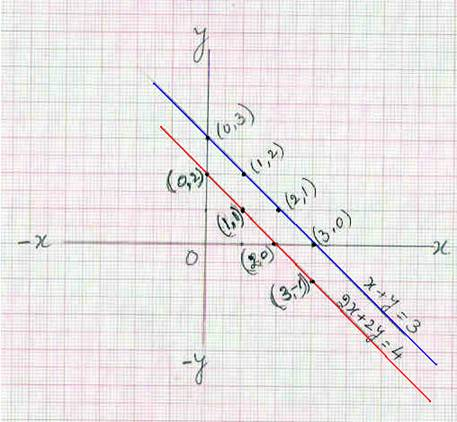7.4 Graphical method of solving Simultaneous linear equations:

Introduction :

We have seen in earlier sections(2.7) that a linear equation is represented a by a line on the graph sheet and hence the name linear equation.

We have also learnt that we need two equations to solve simultaneous liner equations.  From these observations, it becomes  logical that why not represent the linear equations  graphically and then  find a point on the graph sheet which cuts the lines representing the given linear equations.

7.4 Problem 1: Solve 2x-y =3 and x+2y =6 graphically.

Solution:

Consider the equation 2x-y =3 and draw a graph for this line on a graph sheet.

Step 1 : Convert the given  equation in  the form of  y = (i.e. LHS will have only y)

Given equation 2x-y =3

i.e.     -y =3-2x(transposition).  Multiply both sides by -1 to get  y=2x-3

Step 2 : For few values of x (though 2 is enough) get  values of y and record them in a table as shown below:

 x à 0 1 2 3 y à -3 -1 1 3 (x, y) (0,-3) (0,-1) (2,1) (3,3)

Step 3 :  Plot the points represented by (x, y) coordinates  on a graph sheet.

Step 4 : Join the points to get a straight line to represent the line y=2x-3(or 2x-y=3)

Note :

1. Though only two points are enough to draw a straight line, we have calculated more values of (x, y) to indicate that there are many solutions to 2x-y =3.

2. It is also clear from the graph that many coordinates like (0.5,-2) on the

line 2x-y =3 satisfy the given equation.

We need another line crossing this line on the graph so that we get an intersection point .The coordinates of this point will give unique solution to these 2 equations.

Let us draw graph for x+2y = 6(Steps 5 to 7 given below)

Step 5 :  Transpose  x  to get  2y = 6-x i.e.   y = (6-x)/2

Step 6 : For few values of x, calculate corresponding values of y and record them in a table as shown below(repetition  of Step 2):

 x à 0 2 3 4 y à 3 2 1.5 1 (x, y) (0,3) (2,2) (3,1.5) (4,1)Step 7 : Plot the points represented by (x, y) coordinates  on a graph sheet and join them to get a straight line.

Step 8 : Let this and the earlier line meet at  point  P( If the 2 lines don’t meet then extend the 2 lines in both directions so that they meet)

Step 9 : Find the coordinates of P which is (2.4,1.8).

Conclusion: The solution the given equation is x =2.4 and y =1.8

Verfication:

Solve the above 2 equations using elimination method:

2x-y = 3 ====è(1)

x+2y = 6 ====è(2)

Multiply (1) by 2:       4x-2y =6 ====è(3)

-----------

Add (2) & (3)            5x+0 = 12

-----------

i.e.  x= 2.4

By Substituting  2.4 for x in (2).

We get 2.4+2y=6 and on transposition we get 2y =3.6(=6-2.4) i.e. y=1.8

This is exactly the coordinates of point P, which was obtained after drawing the graph

7.4 Problem 2 : Solve 2x+2y = 4 and x+y = 2 graphically

Solution:

Given equation 2x+2y =4

Step 1: (Express the equation as y=) :2y = 4-2x (On transposition)  i.e.   y = 2-x   (On simplification)

Step 2: For few values of x get values of y and record them in a table as shown below:

 x à 0 1 2 3 y à 2 1 0 -1 (x, y) (0,2) (1,1) (2,0) (3,-1)

Step 3: Draw a graph using these (x, y) coordinates to represent the line 2x+2y=4

Step 4: Take 2nd equation x+y =2 i.e.  y =2-x ( On transposition)

Step 5: Since this equation is same as2x+2y=4, The (x,y) coordinate pairs can be obtained from step 2.

 x à 0 1 2 3 y à 2 1 0 -1 (x, y) (0,2) (1,1) (2,0) (3,-1)

Step 6 : Since the coordinates are same as in Step 2, this line is  same as the one that was drawn in step 3(The two lines are collinear)

Conclusion : There are infinite  values of (x, y) which satisfy the given set of equations.

Hence there is no unique solution to the simultaneous liner equations: 2x+2y = 4 and x+y = 2.Verification: Refer 2.14 problem 3.

7.4 Problem 3: Solve 2x+2y = 4 and x+y = 3 graphically

Solution:

Given equation is 2x+2y =4

Step 1: (Express the given equation as y=)i.e.    2y =4-2x (On transposition)

i.e.     y = 2-x   (On simplification))

Step 2: For few values of x calculate values of y and record them in a table as  below:

 x à 0 1 2 3 y à 2 1 0 -1 (x, y) (0,2) (1,1) (2,0) (3,-1)

Step 3: Draw a graph using these (x, y) coordinates to represent the line 2x+2y=4

Step 4: Consider the 2nd equation x+y =3 i.e.  y =3-x (On transposition)

Step 5: For few values of x, calculate values of y and record them in a table as  below: (repetition of Step 2)

 x à 0 1 2 3 y à 3 2 1 0 (x, y) (0,3) (1,2) (2,1) (3,0)

Step 6: Draw a graph using these (x, y) coordinates to represent the line x+y=3

(repetition of step 3)

Step 7 : Since these two lines are parallel, they never meet

Conclusion : There are no values of x and y which satisfy both the given equations:

2x+2y = 4 and x+y =3Verification:  Refer 2.14 problem 4.

Observations: The graphs of

a1 x+ b1 y = c1  and

a2 x+b2 y = c2

Will be

1. Parallel if they have no solution

2. Coincident (concurrent) if they have infinite solutions

3. Intersecting if they have a unique solution

7.4 Problem 4 :  The  cost of manufacturing x articles is Rs 50+3x. The selling price of x articles is 4x. on a graph sheet draw two graphs, first for the cost of manufacturing and second for selling price. From  the graph find out the number of articles to be manufactured and sold to break even(no profit and no loss)

Solution:

The equation for cost of manufacturing articles is CP=3x+50.

The equation for selling price of articles is SP=4x

Using the below mentioned scale, draw two straight lines on a graph sheet representing the above two equations (CP and SP)

x axis for number of articles : 1 cm = 10 articles

y axis for Rupees 1cm = 10Rs

Table for CP

 x à 0 10 20 CP à 50 80 110 (x, y) (0,5) (1,8) (2,11)

Table for SP

 x à 0 10 20 SP à 0 40 80 (x, y) (0,0) (1,4) (2,18)

We note that these two straight lines meet at (5,20)

This means that the cP of  manufacturing 50 articles = SP of 50 articles. Thus the break even happens at the manufacture of 50 articiles

Verification:   if x = 50 then CP = 3x+50=150+50=200

With x=50,  SP = 4x=200

Thus for x=50, CP=SP=Rs 200 and hence our solution is correct.

7.4 Summary of learning

 No Points studied 1 Simultaneous linear equations can also be solved by drawing graph for each of the lines. 2 The intersection of the two lines gives the solution. 3 If the lines are parallel then there is no solution 4 If the lines are concurrent then there are infinite solutions